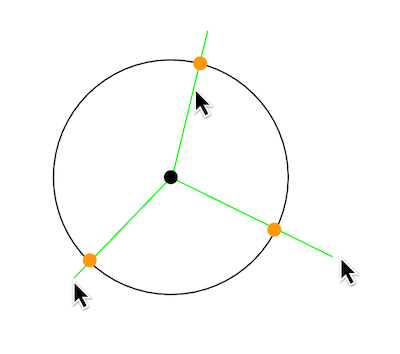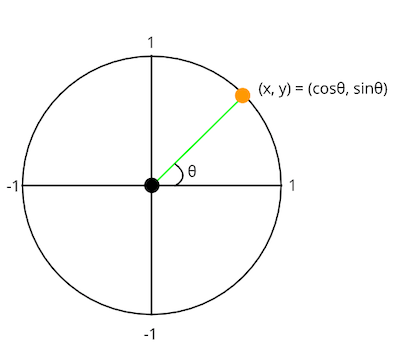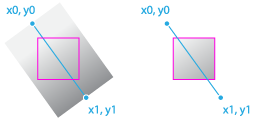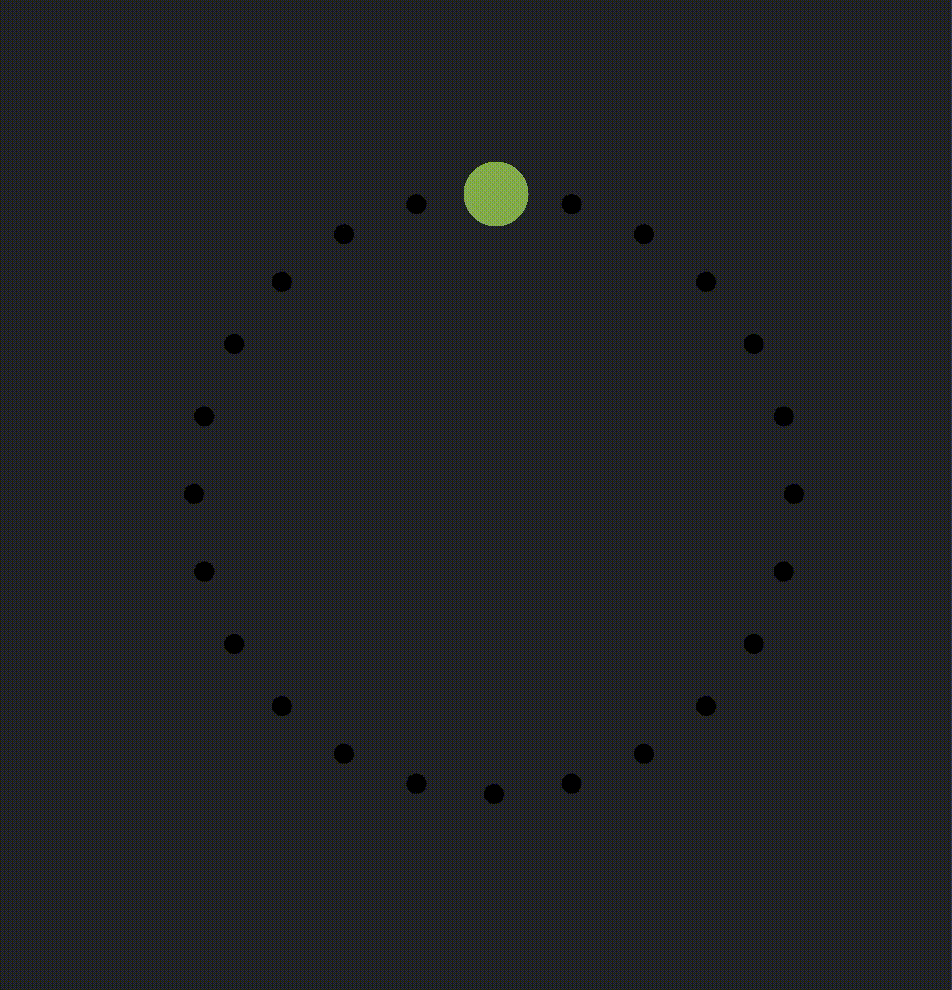# Easy Circle Slider on Canvas without Library## Introduction

The Generative Design Drill Series is all about interactive and generative designs in JavaScript. In this post I implemented a quick and dirty circular slider as shown in the original image below.## How to Calculate Position

I think there are various approaches to achieve to create such an image, but this circular slider draws on a Canvas object. The first difficulty is controlling the slider along the mouse movement. Since normal sliders represented in vertical slider and horizontal slider only need to slide vertically or horizontally, all you have to measure is the y-coordinate or x-coordinate of the mouse movement. However, with this circular slider, it cannot determine where the current cursor is on the slider because the mouse cursor and slider position is not as parallel position as they are. So how should the mouse cursor and slider move together? My methodology is like this.In short, the point, where the straight line connecting the centre point and the mouse cursor and the circle-slider overlap, is regarded as the current slider position. Therefore, the relative slider position does not change whether the mouse cursor is inside or outside the circle-slider. The important thing here is how to find the intersection point. This is a simple trigonometric example, but first consider on how to find a point on the circle. If the radius of the point as (x, y) on the circle is represented by the following formula:

(x, y) = (radius * cosθ, radius * sinθ)If the value of θ is exposed, it can calculate the points on the circle. Recall that the point on the circle is on a straight line with the mouse cursor. Therefore, the desired angle is the same as the angle formed by the centre point and the mouse cursor. Fortunately, the coordinates of the centre point and the coordinates of the mouse cursor can be acquired in advance, so there is information to calculate the angle. Use the following magic function:

const theta = Math.atan2( mouseY — centerY, mouseX — centerX);


This Math.atan2 function calculates the angle in radians based on the x-axis from the x and y coordinates. The following is an excerpt from the MDN reference.

The Math.atan2() function returns the angle in the plane (in radians) between the positive x-axis and the ray from (0,0) to the point (x,y), for Math.atan2(y,x).

The next step is to paint the gradient colour. How can the circle be painted seamlessly? Here is a cheap and cheerful function in canvas to achieve it:

CanvasGradient ctx.createLinearGradient(x0, y0, x1, y1);// NOTE: Set gradient colour for stroke
const canvas = document.getElementById('canvas');
gradient.addColorStop(0, '#758E50');